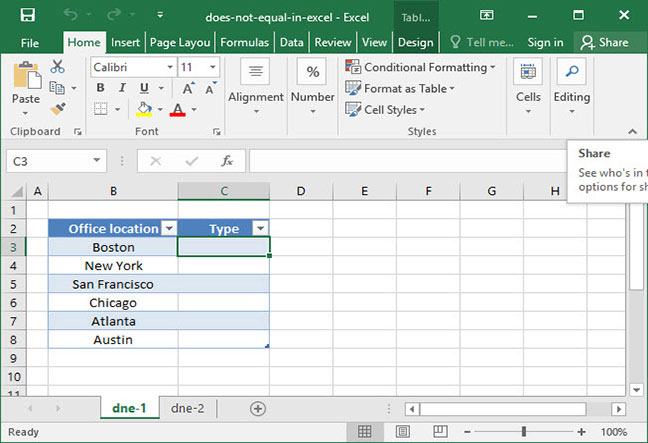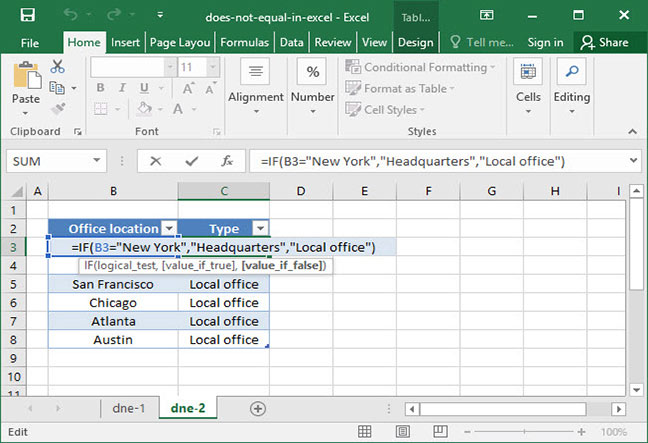# Using the "does not equal" operator in Excel

If you're familiar with logical functions in Excel, you've probably used IF statements to execute different actions based on variable input criteria. In the majority of these scenarios, it's likely that you've used Excel's "=" logical operator to determine whether two values in your formula are equivalent to each other.

But there are some situations in which you may need to figure out whether two values are not equal to one another. This is possible using the NOT function, but there's an even easier way: use the "does not equal" operator to determine whether two statements are not equal. Read on to find out how.

This tutorial will assume that you know how to use Excel's TRUE and FALSE boolean functions. If you're not familiar with those, stop by our TRUE and FALSE tutorials before proceeding.

## The "does not equal" operator

Excel's "does not equal" operator is simple: a pair of brackets pointing away from each other, like so: "<>". Whenever Excel sees this symbol in your formulas, it will assess whether the two statements on opposite sides of these brackets are equal to one another. If they are not equal, it will output TRUE, and if they are equal, it will output FALSE. This is the exact opposite functionality of the equals sign (=), which will output TRUE if the values on either side of it are equal and FALSE if they are not.

Let's take a look at the "does not equal" operator in action to see how we can use it in a simple formula:

`=6<>8Output: TRUE`

The above formula outputs TRUE, because 6 does not equal 8. Let's take a look at another simple example using integers:

`=45<>45Output: FALSE`

This formula outputs FALSE, because 45 is equal to 45.

Of course, "<>" doesn't have to be used on numbers. It can also be used on strings of text. Can you tell why the following formulas output the given results?

`="Boston"<>"San Francisco"Output: TRUE`
`="New York"<>"New York"Output: FALSE`
`=RIGHT("Boston, MA", 2)<>"MA"Output: FALSE`

Hint: For the last example above, you'll have to read up on how the RIGHT function works if you don't already know it!

## Combining <> with IF statements

The "does not equal" operator is useful on its own, but it becomes most powerful when combined with an IF function. Let's take a look at a practical example to see how this works.

The following example uses the IF function. If you haven't used IF statements yet, check out our IF statement tutorial first.The spreadsheet above shows a list of SnackWorld's office locations around the country. The company's headquarters is in New York, and all of the other offices are local. A SnackWorld manager wants to add a column to the spreadsheet that dynamically outputs whether a given office is the company headquarters or a local office.

To do so, we could use the following formula:`=IF(B3<>"New York","Local office","Headquarters")Output: "Local office"`

Note that this formula outputs "Local office" for all the offices names that do not equal "New York"; but, it outputs "Headquarters" when it sees that the office name is equal to "New York".

Note that the above formula could be rewritten as follows, using the equals operator (=) but switching the order of the IF statement's value_if_true and value_if_false arguments:

`=IF(B3="New York","Headquarters","Local office")Output: "Local office"`

Is there any advantage to using the "<>" operator instead of the equals sign? Definitely. When you're using IF statements, you can swap around the order of arguments and generally use either "=" or "<>" in your formulas. But when working with more advanced conditional formulas — in particular, SUMIF and COUNTIF — you'll likely bump into scenarios in which only "<>" is sufficient (for example, if you want to sum up sales for all offices for which the office name is not "New York").

## Other logical operators

If you found this article useful, consider taking a look at our full article on logical operators. We'll teach you how to use the full range of logical operators, including "greater than" and "less than", in your formulas.

## Save an hour of work a day with these 5 advanced Excel tricks

Work smarter, not harder. Sign up for our 5-day mini-course to receive must-learn lessons on getting Excel to do your work for you.

• How to create beautiful table formatting instantly...
• Why to rethink the way you do VLOOKUPs...
• Plus, we'll reveal why you shouldn't use PivotTables and what to use instead...